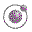The existing LAML make-selector-function. Lecture 4 - slide 5 : 34Program 1
```;; Returns a function, which selects element number n in a list.
;; The second parameter, which is optional, is used for error message purposes.
;; In general, this parameter should be a string corresponding to the name of the selector function.
;; If the second parameter is given, we check whether the list is long enough for selection.
;; If not, we give a decent error message. We recommend use of the second parameter in order to
;; avoid meaningless error messages.
;; The first element is number 1.
;; (make-selector-function 1) corresponds to car, (make-selector-function 2) corresponds to cadr, etc.
;; .form (make-selector-function n [selector-name])
(define (make-selector-function n . optional-parameter-list)
(let ((selector-name (optional-parameter 1 optional-parameter-list #f)))
(if selector-name
(lambda (lst)
(let ((lgt (length lst)))
(if (> n lgt)
(display-error (string-append "The selector function " (as-string selector-name) ": "
"The list "  (as-string lst) " is is too short for selection. "
"It must have at least " (as-string n) " elements."
))
(list-ref lst (- n 1)))))
(lambda (lst) (list-ref lst (- n 1))))))```